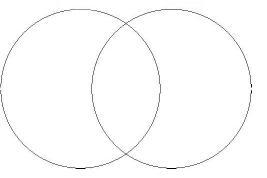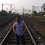# One, Two ....infinityProve that any two circles intersect each other at maximum two points.Note by Writabrata Bhattacharya
6 years, 4 months ago

This discussion board is a place to discuss our Daily Challenges and the math and science related to those challenges. Explanations are more than just a solution — they should explain the steps and thinking strategies that you used to obtain the solution. Comments should further the discussion of math and science.

When posting on Brilliant:

• Use the emojis to react to an explanation, whether you're congratulating a job well done , or just really confused .
• Ask specific questions about the challenge or the steps in somebody's explanation. Well-posed questions can add a lot to the discussion, but posting "I don't understand!" doesn't help anyone.
• Try to contribute something new to the discussion, whether it is an extension, generalization or other idea related to the challenge.

MarkdownAppears as
*italics* or _italics_ italics
**bold** or __bold__ bold
- bulleted- list
• bulleted
• list
1. numbered2. list
1. numbered
2. list
Note: you must add a full line of space before and after lists for them to show up correctly
paragraph 1paragraph 2

paragraph 1

paragraph 2

[example link](https://brilliant.org)example link
> This is a quote
This is a quote
    # I indented these lines
# 4 spaces, and now they show
# up as a code block.

print "hello world"
# I indented these lines
# 4 spaces, and now they show
# up as a code block.

print "hello world"
MathAppears as
Remember to wrap math in $$ ... $$ or $ ... $ to ensure proper formatting.
2 \times 3 $2 \times 3$
2^{34} $2^{34}$
a_{i-1} $a_{i-1}$
\frac{2}{3} $\frac{2}{3}$
\sqrt{2} $\sqrt{2}$
\sum_{i=1}^3 $\sum_{i=1}^3$
\sin \theta $\sin \theta$
\boxed{123} $\boxed{123}$

Sort by:

The statement is wrong: two concentric circles with the same radius intersect at infinitely many points.

Otherwise suppose they are distinct circles. Suppose they intersect at at least three points. Choose three of them, A, B, C. Then ABC has more than 1 circumcircle, a contradiction. Why is this so?

If there were more than 1 circumcircle, then there must be more than one point equidistant to A, B, C. But when a point is equidistant to A and B it must lie on the perpendicular bisector of AB. Similarly it lies on the bisetor of BC. Thus two distinct points both lie on two distinct lines, a contradiction as two distinct lines intersect at at most 1 point!

- 6 years, 4 months ago

Use coordinate geometry.

WLOG, let first circle be expressed as: $S_1:x^2+y^2+2g_1x+2f_1y+c_1=0$

and, second circle as: $S_2:x^2+y^2+2g_1x+2f_1y+c_1=0$

Now, $S_1-S_2=L:2(g_1-g_2)x+2(f_1-f_2)y+(c_1-c_2)=0$

The above is a straight line. If the circles intersect at points $P_1,P_2,P_3,...$, all of these points must and will lie on this line. Thus, we need to solve this line with one of the given circles to get the intersection points. If we substitute for $x$ back into one of the circle equations, it will yield a quadratic equation, which can have at most two distinct real roots. For each distinct real root in $x$, there will be only one real root in y(we get this value by substituting value of $x$ in the line). Hence, two circles can intersect at a maximum of 2 distinct points*.

*The fully intersecting(superimposing) case is a degenerate one which can be understood easily.

- 6 years, 4 months ago

Nice proof through coordinate geometry

- 6 years, 4 months ago

Hint: There is a 2 line solution.

Hint: Any triangle has at most one circumcircle. Prove this in (essentially) 1 line.

Staff - 6 years, 4 months ago

thanks

- 6 years, 4 months ago

That was beautiful.

- 6 years, 4 months ago

Actually yes

- 6 years, 4 months ago

It can also be proved in this way,

Suppose the opposite, and let circle ABC cut the circle DEF at more points than two, that is at B,G,F,H.

refer to this image

Clearly circle DEF will not actually be a circle. For the sake of demonstrating a contradiction, we suppose that it is.

Join BH and BG, and bisect them at K and L.

From K and L, draw perpendiculars to BH and BG, through to A and E.

Since AC cuts BH into two equal parts at right angles, from the porism to Finding Center of Circle, the center of circle ABC is on AC.

For the same reason, the center of circle ABC is also on NO.

But as the only point on both AC and NO is P, it follows that P is the center of circle ABC.

Similarly, we can show that P is also the center of circle DEF.

So we have two circles that intersect which have the same center.

From Intersecting Circles have Different Centers, this statement is contradictory.

Hence such a pair of circles, i.e. those that intersect at more points than two, can not exist.

- 6 years, 4 months ago

If they have to intersect at more than two points, then they have to lie on each other. . .

- 6 years, 4 months ago

Yes...you need to prove that

- 6 years, 4 months ago

I will try to do it later, as I currently have my final exams. Yeah, I've liked your problem!

And yes consider this too, if they have to intersect at more than two points, then they must have the same radius too.

- 6 years, 4 months ago

they will overlap

- 6 years, 4 months ago